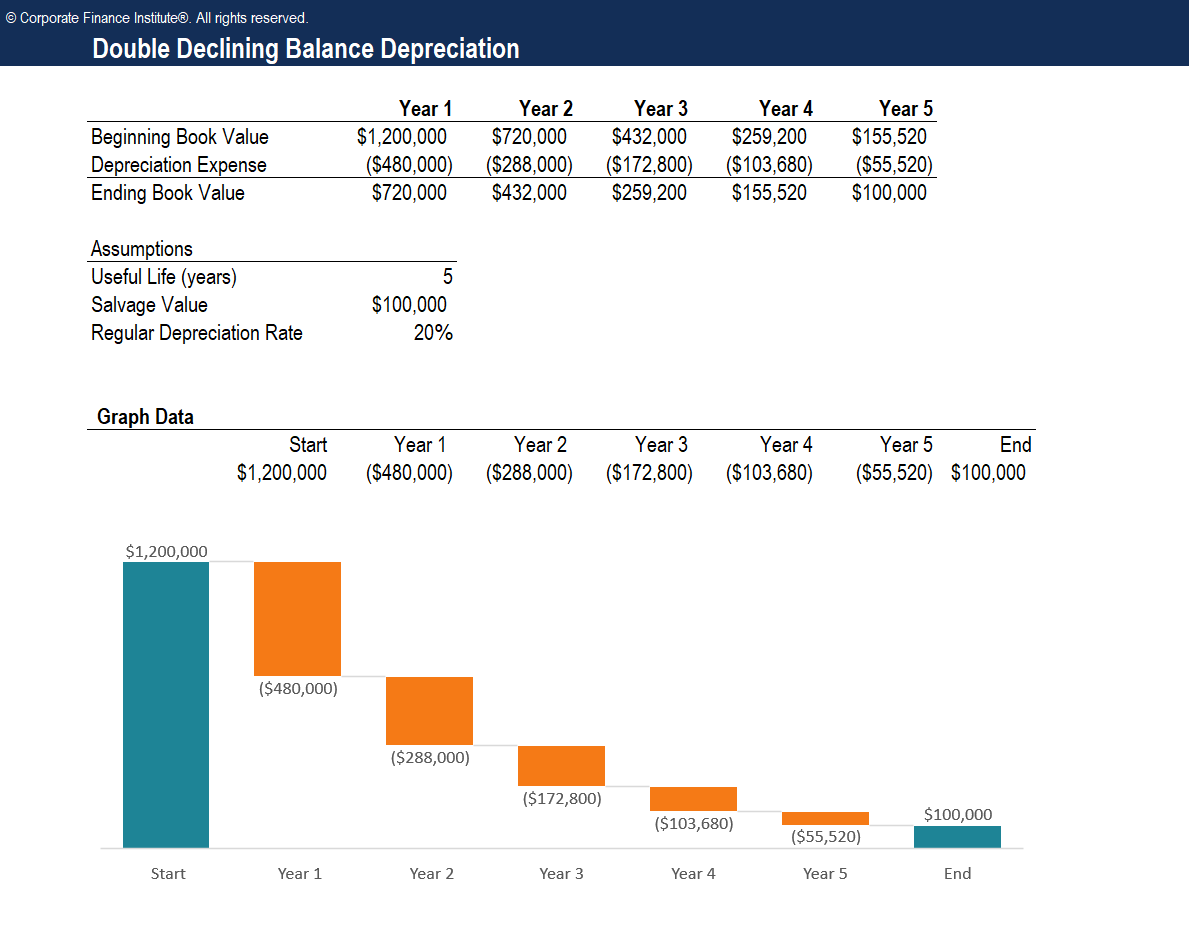Double Declining Balance Depreciation Template

Double Declining Balance Depreciation Template

This double declining balance depreciation template will help you find depreciation expense using one of the most common depreciation methods.

Here is a preview of the template:Enter your name and email in the form below and download the free template now!

How to Calculate the Double Declining Balance

Let’s examine the steps that need to be taken to calculate this form of accelerated depreciation.

Steps:

1. Obtain the beginning book value of the asset (i.e. \$1,200,000).
2. Determine the useful life of the asset (i.e. 5 years).
3. Determine the salvage value of the asset (i.e. \$200,000).
4. Deduct the salvage value from the from the beginning book value to determine the total depreciable amount for the life of the asset (i.e. \$1,200,000 – \$100,000 = \$1,100,000).
5. Calculate the annual depreciation rate (i.e. 100% / 5 years = 20%).
6. Multiply the beginning period book value by the twice the regular annual rate (i.e. \$1,200,000 x 40% = \$480,000).
7. Deduce the annual depreciation expense from the beginning period value to calculate the ending period value.
8. Repeat the above steps until the salvage value is reached.

More Free Templates

For more resources, check out our business templates library to download numerous free Excel modeling, PowerPoint presentation and Word document templates.

• Excel Modeling Templates
• PowerPoint Presentation Templates
• Transaction Document Templates

Financial Analyst Certification

Become a certified Financial Modeling and Valuation Analyst (FMVA)® by completing CFI’s online financial modeling classes and training program!